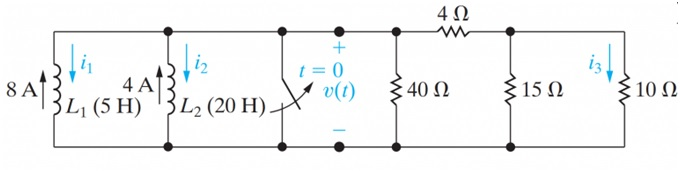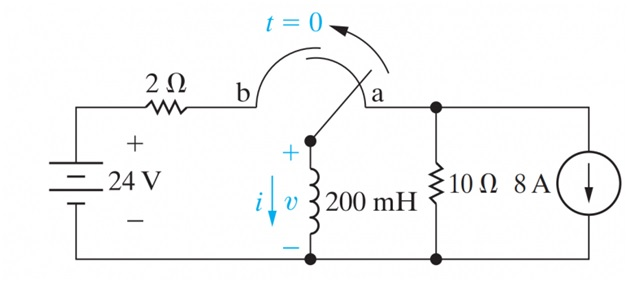### GET ANSWERS FOR ALL ASSIGNMENTS#### Questions 1

Circuit shown in Figure1 is series parallel resistance and inductors, the initial currents in inductors L1 and L2 ​ have been established by sources not shown. The switch is opened at t=0.

1. Find i1i2 and i​for t≥0.
2. Calculate the initial energy stored in the parallel inductors.
3. Determine how much energy is stored in the inductors as t→∞.
4. Show that the total energy delivered to the resistive network equals the difference between the results obtained in (b) and (c).#### Questions 2

The switch in the circuit shown in Figure 2 has been in position a for a long time. At t=0, the switch moves from position a to position b. The switch is a make-before-break type; that is, the connection at position b is established before the connection at position a is broken, so there is no interruption of current through the inductor.

1. Find the expression for (t) for t≥0.
2. What is the initial voltage across the inductor just after the switch has been moved to position b?
3. How many milliseconds after the switch has been moved does the inductor voltage equal 24 V?